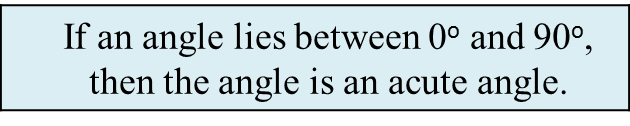# SPM Mathematics 2018, Paper 2 (Questions 5 & 6)

Question 5 (4 marks):
Solving using matrix method is not allowed in this question.
Diagram 5 shows a rectangular fish pond with the perimeter of 62 m.It is given that the length of the fish pond is 3 times its width.
Calculate the length, in m, of the fish pond.

Solution:
Given y + 4 = 3x
y = 3x – 4 …………. (1)

Perimeter = 62
2x + 2(y + 4) = 62
2x + 2y + 8 = 62
2x + 2y = 54
(÷2) x + y = 27 …………. (2)

Substitute (1) into (2):
x + 3x – 4 = 27
4x – 4 = 27
4x = 27 + 4
4x = 31
x = 7.75

From (1): y = 3x – 4
When x = 7.75
y = 3(7.75)4
y = 19.25

Length of the fish pond
= y + 4
= 19.25 + 4
= 23.25 m

Question 6 (5 marks):
(a) State whether the following statement is true or false.(b)
Write down the converse of the following implication:(c)
Write down Premise 2 to complete the following argument:
Premise 1   :  If x is an odd number, then x is not divisible by 2.
Premise 2   : ………………………………………………………
Conclusion : 24 is not an odd number.

(d)
Based on the information below, make one conclusion by deduction for the surface area of sphere with radius 9 cm.Solution:
(a)
True

(b)
If an angle is an acute angle, then the angle lies between 0o and 90o.

(c)
24 is divisible by 2.

(d)
4π(9)2 = 324π
Surface area of the sphere is 324π.